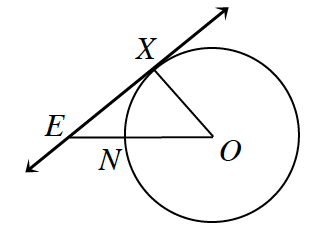### Home > CCG > Chapter Ch10 > Lesson 10.1.5 > Problem10-59

10-59.In the figure at right, $\overleftrightarrow{ E X }$ is tangent to $⊙O$ at point $X$. $OE=20$ cm and $XE=15$ cm.

1. What is the area of the circle?

According to the definition of the tangent line, what is the measure of $∠EXO$?
How can you use this to find the length of $XO$?

A tangent line is perpendicular to a radius, so the measure of $∠EXO = 90º$, so the triangle is a right triangle. So we can use the Pythagorean Theorem.

$\left(XE\right)^2+\left(XO\right)^2=\left(OE\right)^2\\ \quad \ 15^2+(XO)^2=20^2\\ \qquad \qquad \ \ \ XO^2=175\\ \qquad \qquad \quad \ XO=13.23$

$A = \pi r^2\\ A=\pi (13.23)^2\\ A = 175\pi\text{ cm}^2\\ A = 549.78\text{ cm}^2$

1. What is the area of the sector bounded by $\overline{OX}$ and $\overline{ON}$?

Find the measure of $∠XON$. Use this to find the area of the sector.

$A\approx74.21\text{ cm}^2$

2. Find the area of the region bounded by $\overline{XE}$, $\overline{NE}$, and $\overarc{ N X }$.

Find the area of the triangle formed by $E, X,\text{ and }O$. Subtract the area of the sector from the area of the triangle.